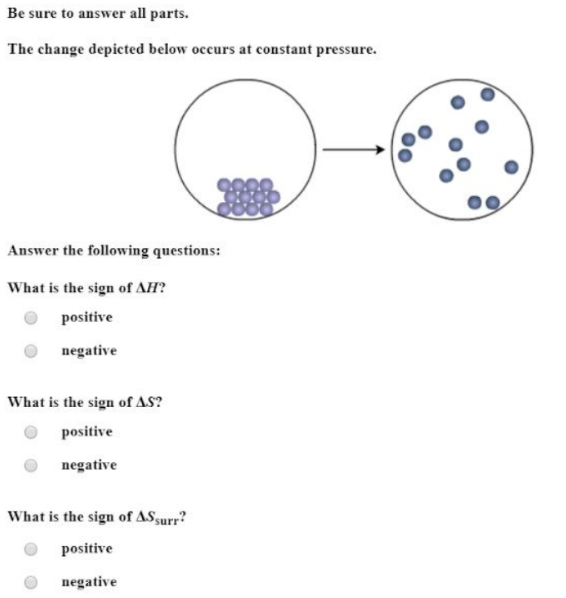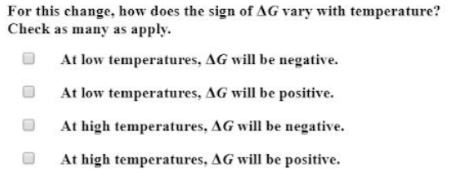# The change depicted below occurs at constant pressure. Answer the following questions: What is the sign of delta H? a. positive b. negative What is the sign of delta S? a. positive b. negative What is the sign of delta S_surr? a. positive b. negative For this change, how does the sign of delta G vary with temperature? Check as many as apply. a. At low temperatures, delta G will be negative. b. At low temperatures, delta G will be positive. c. At high temperatures, delta G will be negative. d. At high temperatures, delta G will be positive.